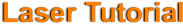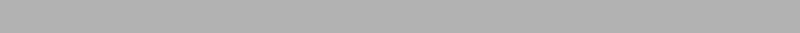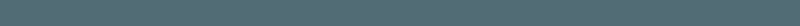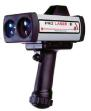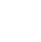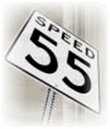The information presented here is intended to  present the basic
principles for understanding of how a Laser device is used to
determine the speed of an object.

Lidar is the name given to Laser speed measurement devices. It simply means,
Light Detection And Ranging. Lidar uses the principle of  Time &  Distance
to determine Speed. The lidar instrument transmits pulse of  light out to a target.
This transmitted light pulse is reflected back to the instrument where it is analysed.
The time for the light to travel out to the target and back to the lidar is used to
determine the range to the target. A second Second Pusle is sent out to the target
and the Time and Distance is then calculated and averaged with the first and the
Speed is thus determined.(simplified for understanding)

Example of distance measurement:
assume the speed of light is c = 3.0E8 m/s
Traveling at the speed of Light, in 1 microsecond you would travel 492 feet.

Average Speed = Distance traveled
Time Taken